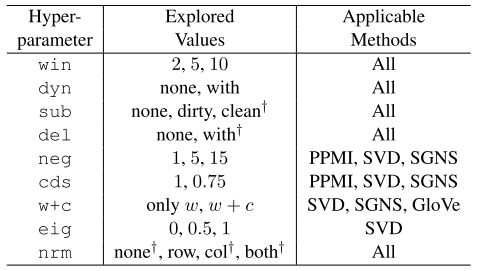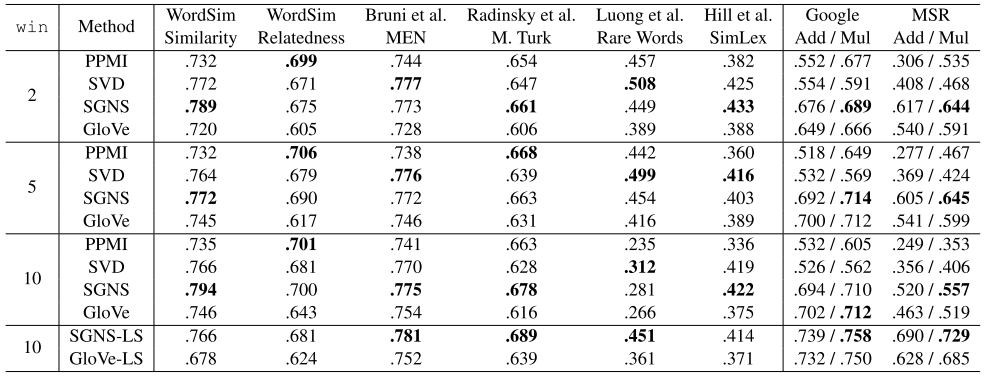# Improving Distributional Similarity with Lessons Learned from Wrod Embeddings

## WHY?

Word embedding using neural network(Skipgram) seems to outperform traditional count-based distributional model. However, this paper points out that current superiority of word2vec is not because of the algorithm itself, but because of system design choices and hyperparameter optimizations.

## Note

Traditional method of word representation is count-based representation (bag-of-contexts). This method is counting the cooccurance of two words within a certain window forming sparse matrix M with row of vocabulary and column of context. The popular measure of assiciation is pointwise mutual information(PMI). To solve the problem of negative values in PMI, modified positive PMI(PPMI) is widely used.

PMI(w, c) = \log\frac{\hat{P}(w,c)}{\hat{P}(w)\hat{P}(c)} = \log\frac{\#(w,c)\cdot|D|}{\#(w)\cdot\#(c)}\\
PPMI(w,x) = max(PMI(w,c), 0)

Since word representation of count-based model can be too sparse, Singular Value Decomposition(SVD) is used on PPMI matrix to reduce the dimension.

M_d = U_d\cdot\Sigma_d\cdot V_d^T\\
W^{SVD} = U_d\cdot\Sigma_d\\
C^{SVD} = V_d

## WHAT?

Author compared PPMI, SVD, SGNS(Skip-gram with negative sampling), and GloVe varing different hyperparameters. This paper defined three kinds of parameters: pre-processing hyperparameters, association metric hyperparameters, and post-processing hyperparameters. Dynamic context window(dyn), subsampling(sub), and deleting rare words(del) are included as pre-processing hyperparameters. Shifted PMI(neg) and context distribution smoothing(cds) are association metric hyperparameters. Adding context vectors(w+c), eigenvalue weighting(eig) and vector normalization(nrm) are post-processing hyperparameters. The space of hyperparameters are as follows.This paper enumerated all the possible hyperparameter space for each embedding method. The quality of embedding was tested on the two kinds of task. Word similarity was tested on WordSim Similarity, WordSim Relatedness, MEN, Mechanical Turk, Rare Words, and SimLex-999 dataset. Word analogy was tested on MSR and Google’s analogy dataset on two different measures: 3CosAdd and 3CosMul.

## So?The most important result was that no single algorithm outperformed the others. The performance was varied by datasets and hyperparameter configuration. Beneficial configurations and practical recommendations were given in the paper.

## Critic

Deep analysis on word embedding. Amazing experiments.

Powered by aiden